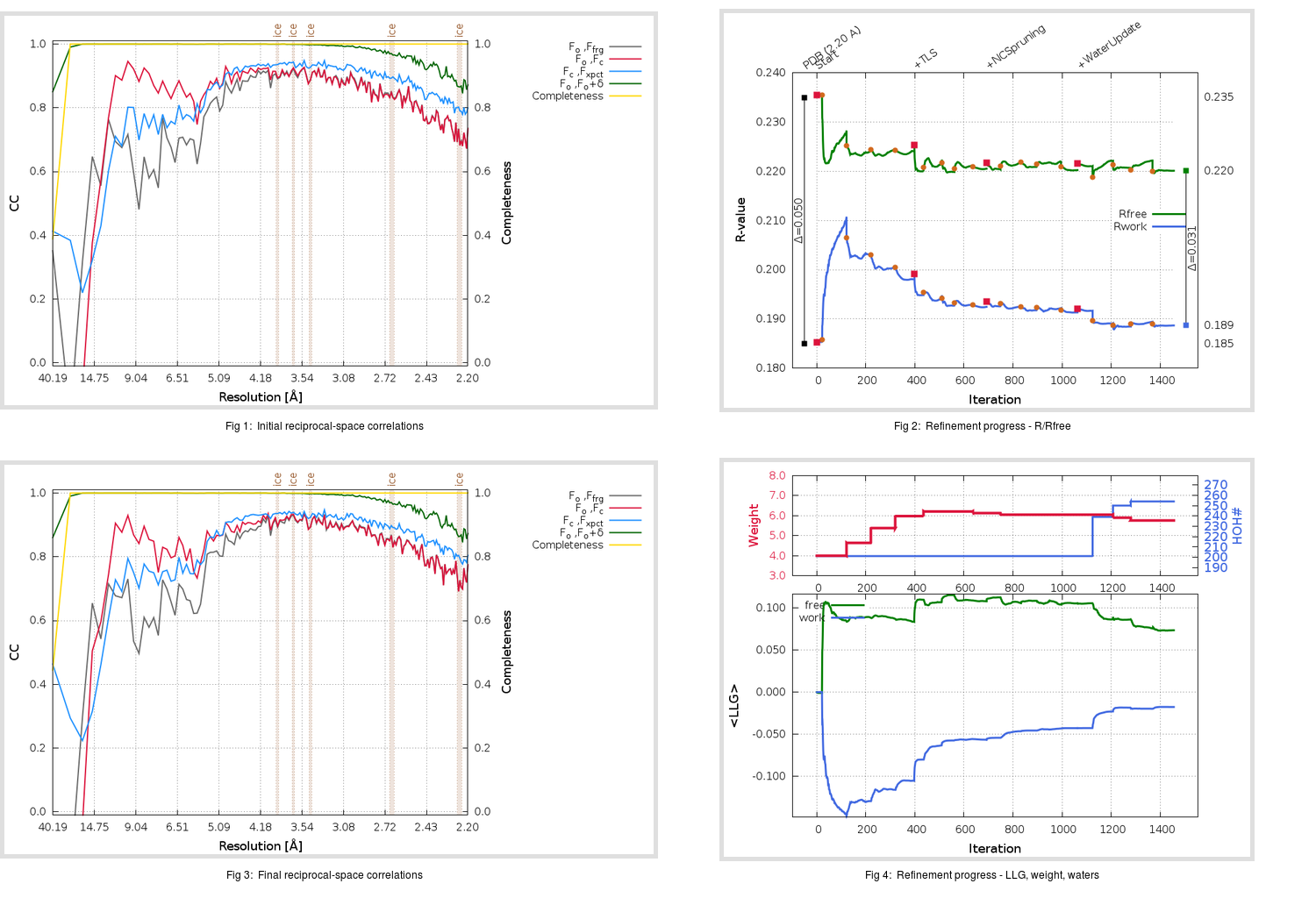Content:

```    Diffraction limits & principal axes of ellipsoid fitted to diffraction cut-off surface:
2.143         0.9996   0.0000  -0.0293       0.950 a* - 0.314 c*
2.164         0.0000   1.0000   0.0000       b*
2.158         0.0293   0.0000   0.9996       0.024 a* + c*
```

## Deposited

` `
 Date deposited Date data collection Resolution R, Rfree 20111231 20090918 2.20 0.1820 0.2350

Molprobity (CCP4 7.0 version) summary:

```Ramachandran outliers =   0.17 %
favored =  95.87 %
Rotamer outliers      =   1.52 %
C-beta deviations     =     2
Clashscore            =   5.32
RMS(bonds)            =   0.0092
RMS(angles)           =   1.11
MolProbity score      =   1.71
Resolution            =   2.20
R-work                =   0.1820
R-free                =   0.2350
```

```Number of waters      =   201

<B> (all atoms) =   35.91 ( sd =   12.21 ) for       5027 non-hydrogen atoms
<B>   (protein) =   35.70 ( sd =   12.37 ) for       4756 non-hydrogen atoms
<B>     (water) =   38.56 ( sd =    7.83 ) for        201 non-hydrogen atoms
<B>    (others) =   41.97 ( sd =    8.80 ) for         70 non-hydrogen atoms

B min/max       (all non-hydrogen atoms) =   16.85 /   95.99
B min/max   (protein non-hydrogen atoms) =   16.85 /   95.99
B min/max     (water non-hydrogen atoms) =   19.50 /   58.95
B min/max     (other non-hydrogen atoms) =   29.46 /   66.13
```

## BUSTER (re-)refinement

` `

Molprobity (CCP4 7.0 version) summary:

```Ramachandran outliers =   0.00 %
favored =  97.69 %
Rotamer outliers      =   2.27 %
C-beta deviations     =     0
Clashscore            =   4.48
RMS(bonds)            =   0.0126
RMS(angles)           =   1.61
MolProbity score      =   1.56
Resolution            =   2.20
R-work                =   0.1887
R-free                =   0.2201
```

```Number of waters      =   254

<B> (all atoms) =   41.18 ( sd =   13.20 ) for       5080 non-hydrogen atoms
<B>   (protein) =   40.60 ( sd =   13.10 ) for       4756 non-hydrogen atoms
<B>     (water) =   48.75 ( sd =   12.35 ) for        254 non-hydrogen atoms
<B>    (others) =   52.84 ( sd =    6.93 ) for         70 non-hydrogen atoms

B min/max       (all non-hydrogen atoms) =   21.67 /  113.11
B min/max   (protein non-hydrogen atoms) =   21.67 /  105.18
B min/max     (water non-hydrogen atoms) =   24.00 /  113.11
B min/max     (other non-hydrogen atoms) =   40.82 /   65.54
```

Refinement progression:Results:

` `
 File Remark 3VB4_aB_refine.01_04_refine.pdb.gz exact refinement commands are in header 3VB4_aB_refine.01_04_refine.mtz.gz including original deposited data and several re-refinement map coefficients 3VB4_aB_refine.01_04_BUSTER_model.cif.gz including any non-standard compound restraints 3VB4_aB_refine.01_04_BUSTER_refln.cif.gz